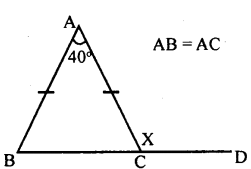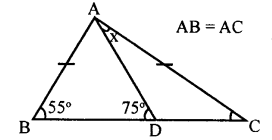# KSEEB Solutions for Class 8 Maths Chapter 11 Congruency of Triangles Ex 11.3

Students can Download Maths Chapter 11 Congruency of Triangles Ex 11.3 Questions and Answers, Notes Pdf, KSEEB Solutions for Class 8 Maths helps you to revise the complete Karnataka State Board Syllabus and score more marks in your examinations.

## Karnataka Board Class 8 Maths Chapter 11 Congruency of Triangles Ex 11.3

Question 1.
In a Δ ABC, AB = AC andlA.= 50° find ∠B and ∠C.
∠A+ ∠B + ∠C = 180°
(Sum of the angles of a triangle is 180° )
50 +∠[B + ∠B = 180°
∠B = ∠C Base angles of an isosceles triangle
50 + 2∠B= 180°
2∠B = 180 – 50
2∠B = 130°
∠B = $$\frac{130^{\circ}}{2}$$
∠B = 65°
∠B = ∠C = 65°

Question 2.
In AABC,AB = BCand|B = 64°find|£,
AB = BC [data]
∴ ∠C = ∠A [Theorem 1]
∠A + ∠B + ∠C = 180°
(Sum of the angles of a triangle is 180°)
∠C + 64 + ∠C = 180° [∠A = ∠C]
64 + 2∠C = 180°
2∠C = 180 – 64
2∠C = 116
∠C = $$\frac { 116 }{ 2 }$$ = 58°

Question 3.
In each of the following figure find the value of x :
i.In ∆ABC, AB = AC
∴ ∠ABC = ∠ACB
∴ ∠BAC + ∠ABC + ∠ACB = 180°
(Sum of the angles of a triangle is 180°)
40 + ∠ABC + ∠ABC = 180°
(∠ABC =∠ACB)
40 + 2∠ABC = 180°
2∠ABC = 180 – 40
2∠ABC = 140°
∠ABC = 70°
∠ACB = ∠ ABC = 70°
∠ACB + ∠ACD = 180°
70 + x = 180°
x = 180 – 70
x = 110°

iiAC = CD
∠ACD + ∠CAD + ∠CDA = 180°
(Sum of the angles of a triangle is 180°)
∠ACD + 30 + 30=180°
∠ACD + 60=180°
∠ACD = 180-60
∠ACD = 120°
∠ACD = ∠BAC + ∠ABC
120 = 65° + x
120 – 65 = x
55 = x
x=55°

iiiiAB = AC
∠ABC = ∠ACB = 55° [Theorem l]
Exterior ∠APB = ∠DAC +∠ACD
75 = x + 55
75 – 55 = x
20 = x
x = 20°

iv.BD = DC = AD & ∠ABD = 50 °
50 + 50 +∠ADB = 180°
∠ADB = 180 – 100 = 80°
∠APB = 80°
∴∠DAC = ∠DCA = x°
∴ x + x +∠ADC = 180°
2x + 100 = 180°
2x = 180-100=80°
x=40°

Question 4.
Suppose ABC is an equilateral triangle. Its base BC is produced to D such that BC = CD.
Calculate: l.∠ACD
∠ABC = ∠ACB = ∠BAC = 60°
(ABC is an equilateral triangle)
∠ACB +∠ACD = 180° (Linearpoint)
60 + ∠ACD = 180°
∠ACD = 180 – 60
∠ACD = 120°In ∆ACD,AC=CD
∠ CAD = ∠CPA (Theorem l)
∠ACB +∠ACD = 180° [linear pair]
60° +∠ACD = 180°
∠ACD = 180° – 60° = 120°
∠ACA +∠CAD + ∠CDA = 180°
2∠CDA = 180 – 120°
2∠CDA = 60°
∠CDA = $$\frac { 60 }{ 2 }$$ = 30°
∠CDA = 30

Question 5.
Show that the perpendicular drawn from the vertices of the base of an isosceles triangle to the opposite sides are equal. ,
Data : In ∆ABC, AB = AC,
BD ⊥AC & CE⊥ AB
To prove : BD = CEProof:
In ∆ABC, AB = AC [data]
∠ABC = ∠ACB [Theorem l]
In ∆EBC and ∆DCB
∠EBC =∠DCB( Base angles )
∠BEC = ∠CDB [= 90° ]
BC = BC (Common side)
∆EBC = ∆DCB [ASA postulate]
BD = CE [Corresponding sides]

Question 6.
Prove that an ∆ABC is an isosceles triangle if the altitude AD from A on BC bisects BC.BD = DC [AD bisects BC]
∴AB = AC [Correspondingsides]
∴∆ ABC is an isosceles triangle

Question 7.
Suppose a triangle is equilateral, prove that it is equiangular.